Computer Science

# While loopsand you want to fill the bottom row with green squares:You might imagine writing code like this:

``````from byubit import Bit

@Bit.empty_world(5, 3)
def go_green(bit):
bit.paint('green')
bit.move()
bit.paint('green')
bit.move()
bit.paint('green')
bit.move()
bit.paint('green')
bit.move()
bit.paint('green')

if __name__ == '__main__':
go_green(Bit.new_bit)``````

If you repeat `bit.paint('green')` and `bit.move()` enough times, you will eventually paint the bottom.

But what if your world was 100 squares wide?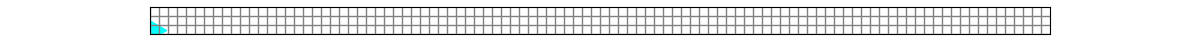The approach above would get really tedious!

## While

This motivates why Python (and other programming languages) have a `while` loop:

``````def go_green(bit):
while bit.front_clear():
bit.paint('green')
bit.move()``````

This code says while the front of bit is clear, paint green and move forward one square.

We are introducing a new Bit function here:

``bit.front_clear()``

This function returns `True` if the front of bit is clear. This will return `True` as long as the space in front of Bit is clear, otherwise it will retur `False`.

Let’s look at the `while` loop more closely:• it must start with the `while` keyword
• this is followed by a condition that evaluates to True or False
• the first line ends with a colon `:`
• the body of the while loop is indented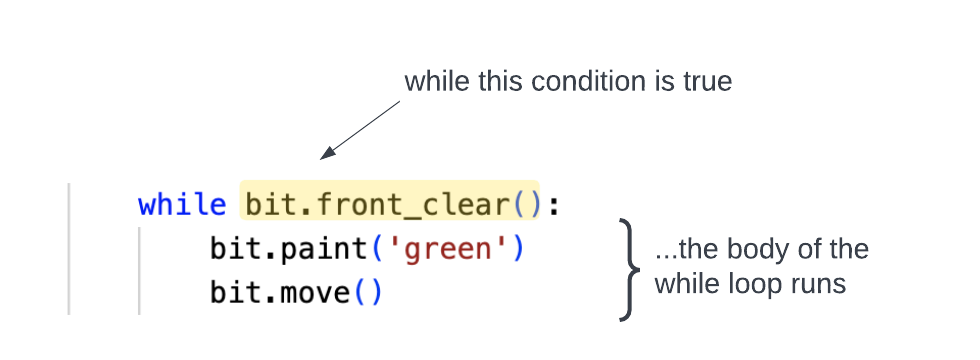Every time Python runs the while loop it checks the condition. If it is true, then it runs the lines of code in the body of the loop. After it runs the body, it checks the condition again. It will keep checking the condition and run the body every time the condition is true. It leaves the loop if the condition is false.

## While in action

To practice with while loops, download the zip file linked above. Find the `go_green.py` file, which looks like this:

``````from byubit import Bit

@Bit.empty_world(5, 3)
def go_green(bit):
while bit.front_clear():
bit.paint('green')
bit.move()

if __name__ == '__main__':
go_green(Bit.new_bit)``````

Run it, and you should see this result:• click the `First` button
• click the `Next` button repeatedly and watch the code execute

You should see Python check if the front is clear. This will be True the first time, so it will paint and then move. It will check the condition again … and repeat the loop, until it checks the condition and finds that the front is not clear, so it stops.

## Boundary conditions

Every time you use a `while` loop, you should think about the boundary conditions:

• Where is Bit when the loop starts?
• Where will Bit be when the loop ends?
• What squares will be colored?
• Which squares will not be colored?
• Which direction is Bit facing at the beginning? at the end?

In the code above, when Bit finishes the while loop, the last square is left unpainted.

### Painting the last square

Remember, we want the whole bottom row to be green. How do we paint the last square green?

You need to add an extra `bit.paint('green')` outside the while loop:

``````def go_green(bit):
while bit.front_clear():
bit.paint('green')
bit.move()

bit.paint('green')``````

Because this last `bit.paint('green')` is not indented, it is outside the while loop. When `bit.front_clear()` is `False`, then Python will skip the loop and go to the next non-indented line. So it will paint one more square green and then finish the `go_green()` function: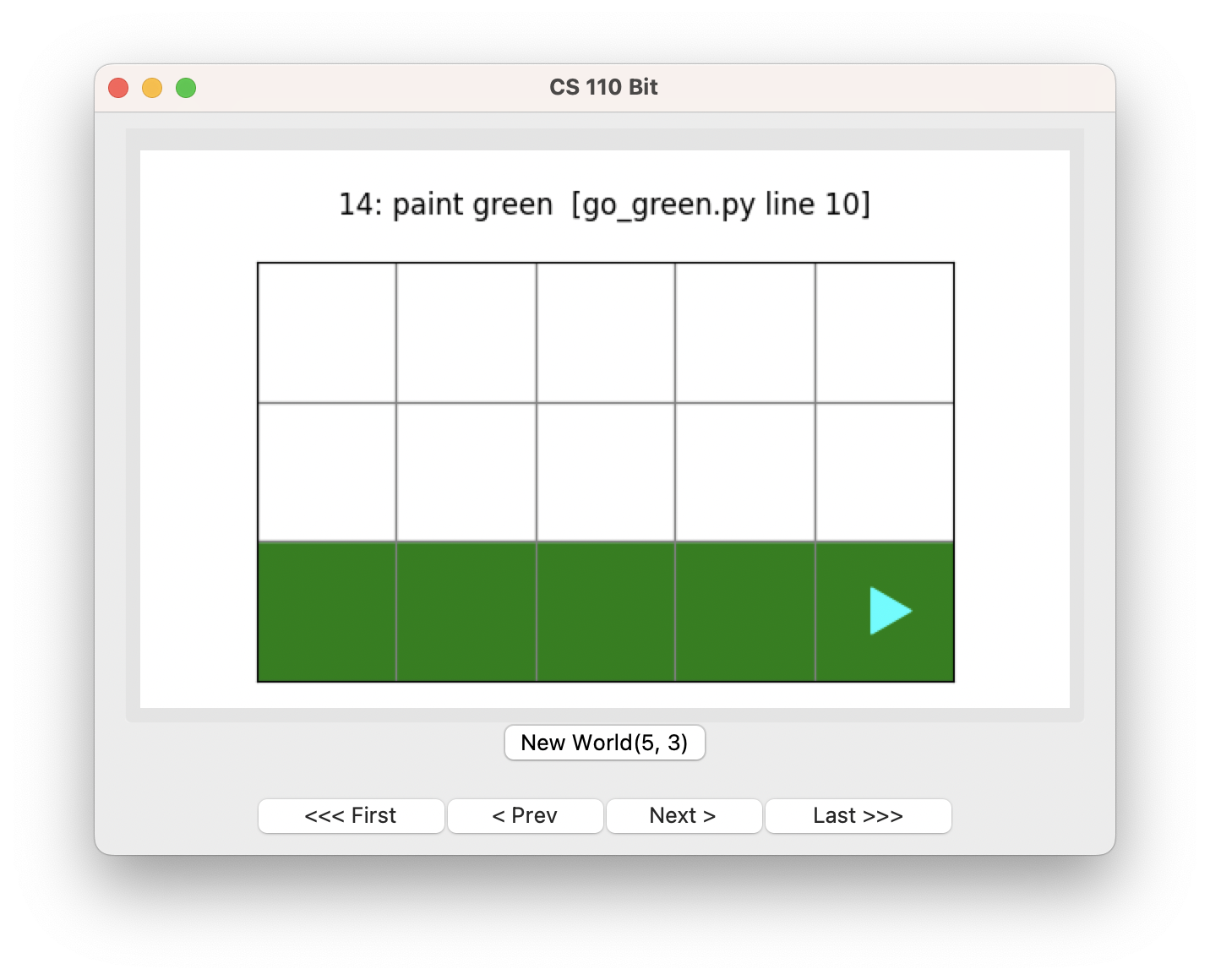### Moving first and then painting

You could also change your `go_green()` function to move first and then paint green:

``````def go_green(bit):
while bit.front_clear():
bit.move()
bit.paint('green')``````

Here, the boundary conditions will be different! This will paint every square green except the first one. Step through the code using the `First` and `Next` buttons to see why.You can paint the entire bottom row green by placing an extra `bit.paint('green')` that comes before the while loop:

``````def go_green(bit):
bit.paint('green')
while bit.front_clear():
bit.move()
bit.paint('green')``````

It’s up to you to decide whether to paint first or move first. There is often more than one way to solve a problem.

## Blocks

Bit worlds may sometimes include black squares: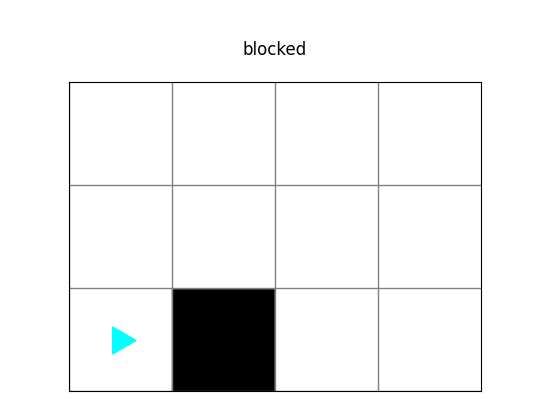Black squares stop bit from moving onto them. To see this in action, open the file called `blocked.py`, which is in the zip file you downloaded:

``````from byubit import Bit

@Bit.worlds('blocked')
def blocked(bit):
bit.move()

if __name__ == '__main__':
blocked(Bit.new_bit)``````

All bit does here is try to move one square. But if you run the code you will see this error: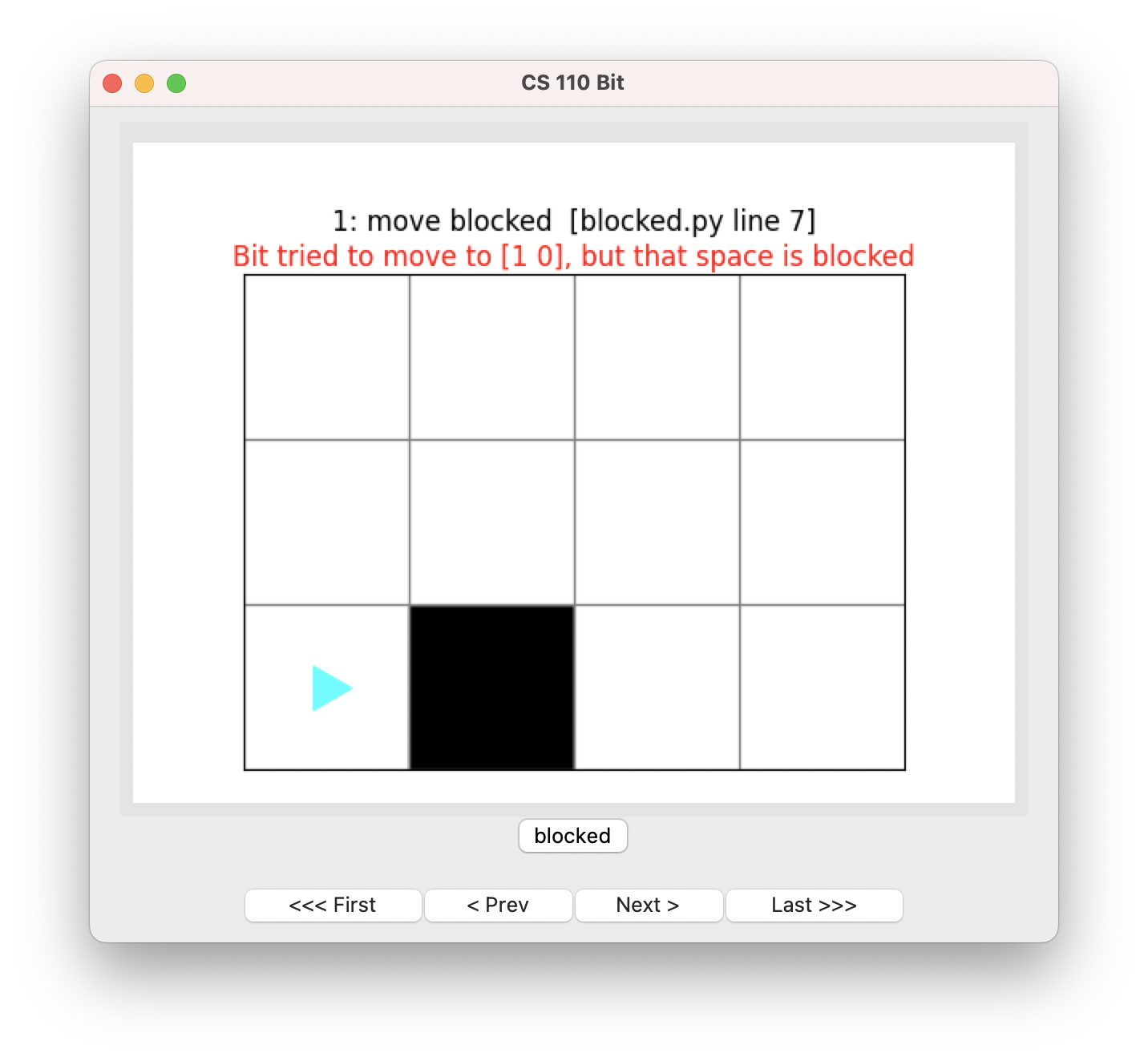## Conditions in Bit

Bit supports the following functions that check whether it is safe to move:

• `bit.front_clear()` — returns `True` if the front is clear, `False` otherwise
• `bit.right_clear()` — returns `True` if the right is clear, `False` otherwise
• `bit.left_clear()` — returns `True` if the left is clear, `False` otherwise

For a square to be clear, it must not be black or the edge of the world.

Looking at this world:• `bit.front_clear()` is `False`
• `bit.right_clear()` is `False`
• `bit.left_clear()` is `True`

Look at the file called `black_row.py`, which is in the zip file you downloaded:

``````from byubit import Bit

@Bit.worlds('black-row')
def go(bit):
while bit.right_clear():
bit.move()

if __name__ == '__main__':
go(Bit.new_bit)``````

Bit starts in this world:We want to move Bit until it is next to the first black square:The code above uses `while bit.right_clear()` to move until the right is no longer clear. Run the code above and use the `First` and `Next` buttons to see how it uses this condition.

## Negating conditions

You can negate conditions using the `not` keyword. For example:

``````while not bit.right_clear()
bit.move()``````

will move Bit as long as the right side is not clear.

To see this in action, open the file called `another-black-row.py`, which is in the zip file you downloaded:

``````from byubit import Bit

@Bit.worlds('another-black-row')
def go(bit):
while not bit.right_clear():
bit.move()

if __name__ == '__main__':
go(Bit.new_bit)``````

Bit starts in this world:We want to move Bit until it gets past all of the black squares:The code above uses `while not bit.right_clear()` to move until the right is clear. Run the code and use the `First` and `Next` buttons to see how it uses this condition.

Python will check `bit.right_clear()` and this will be `False`. But then the `not` turns the `False` to `True`.

At the end of the loop, Python will check `bit.right_clear()` and this will be `True`. But then the `not` turns the `True` to `False`, and so Bit leaves the while loop.

## More conditions in Bit

Bit also provides a way for you to check which color of square Bit is currently on top of:

• `bit.is_blue()` — returns `True` if Bit is on a blue square
• `bit.is_green()` — returns `True` if Bit is on a green square
• `bit.is_red()` — returns `True` if Bit is on a red square
• `bit.is_empty()` — returns `True` if Bit is on an empty square (no color)

To see this in action, open the file `green_path.py`, which is in the zip file you downloaded:

``````from byubit import Bit

@Bit.worlds('green-path')
def walk(bit):
while bit.is_green():
bit.move()

if __name__ == '__main__':
walk(Bit.new_bit)``````

Bit starts in this world:We want to have Bit follow the green squares until it gets past the last one:The code above uses `while bit.is_green()` to move until the square it is on is not green. Run the code and use the `First` and `Next` buttons to see how it uses this condition.

You can find another example in `blue_dot.py`:

``````from byubit import Bit

@Bit.worlds('blue-dot')
def go_to_blue(bit):
while not bit.is_blue():
bit.move()

if __name__ == '__main__':
go_to_blue(Bit.new_bit)``````

Bit starts in this world:We want to have Bit move until it gets to the blue square:The code above uses `while not bit.is_blue()` to move until the square it is on is blue. Run the code and use the `First` and `Next` buttons to see how it uses this condition.

## Infinite loops

Open the file called `infinite_loopy.py` in the zip file you downloaded:

``````from byubit import Bit

@Bit.empty_world(3, 3)
def run(bit):
while bit.front_clear():
bit.move()
bit.paint('blue')
bit.move()
bit.paint('blue')
bit.left()

if __name__ == '__main__':
run(Bit.new_bit)``````

This code works on an empty 3x3 world: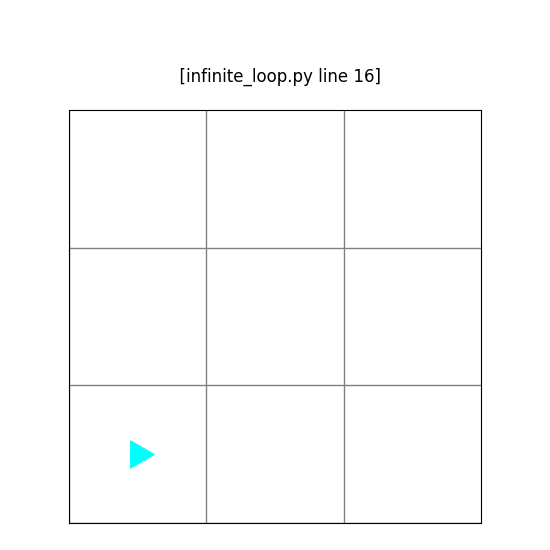In the code, while the front is clear, bit will:

• move
• paint a blue square
• move
• paint a blue square
• turn left

You can draw this out and see that Bit will paint the bottom row blue and then turn left: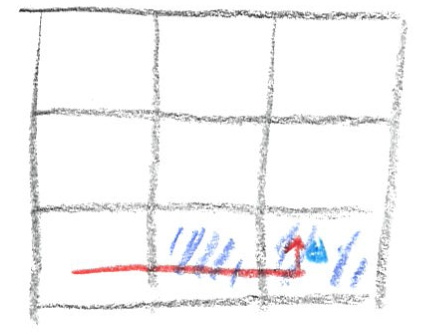Since the front of bit is clear, it will do this again:and again and again and again …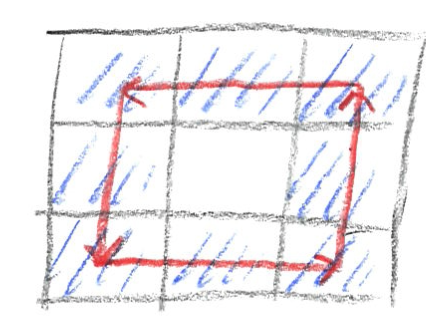Thankfully, if you run the code, Bit will alert you if you have created an infinite loop: